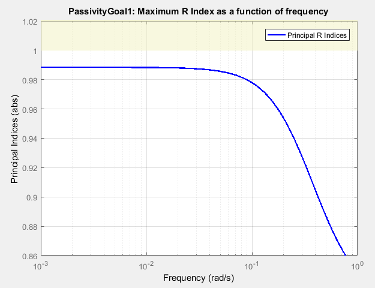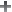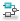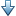## Passivity Goal

### Purpose

Enforce passivity of specific input/output map when using Control System Tuner.

### Description

Passivity Goal enforces passivity of the response of the transfer function between the specified signal locations. A system is passive if all its I/O trajectories (u(t),y(t)) satisfy:

`${\int }_{0}^{T}y{\left(t\right)}^{T}u\left(t\right)dt>0,$`

for all T > 0. Equivalently, a system is passive if its frequency response is positive real, which means that for all ω > 0,

`$G\left(j\omega \right)+G{\left(j\omega \right)}^{H}>0$`

Passivity Goal creates a constraint that enforces:

`${\int }_{0}^{T}y{\left(t\right)}^{T}u\left(t\right)dt>\nu {\int }_{0}^{T}u{\left(t\right)}^{T}u\left(t\right)dt+\rho {\int }_{0}^{T}y{\left(t\right)}^{T}y\left(t\right)dt,$`

for all T > 0. To enforce the overall passivity condition, set the minimum input passivity index (ν) and the minimum output passivity index (ρ) to zero. To enforce an excess of passivity at the inputs or outputs, set ν or ρ to a positive value. To permit a shortage of passivity, set ν or ρ to a negative value. See About Passivity and Passivity Indices for more information about these indices.In Control System Tuner, the shaded area on the plot represents the region in the frequency domain in which the tuning goal is not met. The plot shows the value of the index described in Algorithms.

#### Creation

In the Tuning tab of Control System Tuner, select New Goal > Passivity Goal.

#### Command-Line Equivalent

When tuning control systems at the command line, use `TuningGoal.Passivity` to specify a passivity constraint.

### I/O Transfer Selection

Use this section of the dialog box to specify the inputs and outputs of the transfer function that the tuning goal constrains. Also specify any locations at which to open loops for evaluating the tuning goal.

• Specify input signals

Select one or more signal locations in your model as inputs to the transfer function that the tuning goal constrains. To constrain a SISO response, select a single-valued input signal. For example, to constrain the gain from a location named `'u'` to a location named `'y'`, clickAdd signal to list and select `'u'`. To constrain the passivity of a MIMO response, select multiple signals or a vector-valued signal.

• Specify output signals

Select one or more signal locations in your model as outputs of the transfer function that the tuning goal constrains. To constrain a SISO response, select a single-valued output signal. For example, to constrain the gain from a location named `'u'` to a location named `'y'`, clickAdd signal to list and select `'y'`. To constrain the passivity of a MIMO response, select multiple signals or a vector-valued signal.

• Compute input/output gain with the following loops open

Select one or more signal locations in your model at which to open a feedback loop for the purpose of evaluating this tuning goal. The tuning goal is evaluated against the open-loop configuration created by opening feedback loops at the locations you identify. For example, to evaluate the tuning goal with an opening at a location named `'x'`, clickAdd signal to list and select `'x'`.

Tip

To highlight any selected signal in the Simulink® model, click. To remove a signal from the input or output list, click. When you have selected multiple signals, you can reorder them usingand. For more information on how to specify signal locations for a tuning goal, see Specify Goals for Interactive Tuning.

### Options

Use this section of the dialog box to specify additional characteristics of the passivity goal.

• Minimum input passivity index

Enter the target value of ν in the text box. To enforce an excess of passivity at the specified inputs, set ν > 0. To permit a shortage of passivity, set ν < 0. By default, the passivity goal enforces ν = 0, passive at the inputs with no required excess of passivity.

• Minimum output passivity index

Enter the target value of ρ in the text box. To enforce an excess of passivity at the specified outputs, set ρ > 0. To permit a shortage of passivity, set ρ < 0. By default, the passivity goal enforces ρ = 0, passive at the outputs with no required excess of passivity.

• Enforce goal in frequency range

Limit the enforcement of the tuning goal to a particular frequency band. Specify the frequency band as a row vector of the form `[min,max]`, expressed in frequency units of your model. For example, to create a tuning goal that applies only between 1 and 100 rad/s, enter `[1,100]`. By default, the tuning goal applies at all frequencies for continuous time, and up to the Nyquist frequency for discrete time.

• Apply goal to

Use this option when tuning multiple models at once, such as an array of models obtained by linearizing a Simulink model at different operating points or block-parameter values. By default, active tuning goals are enforced for all models. To enforce a tuning requirement for a subset of models in an array, select Only Models. Then, enter the array indices of the models for which the goal is enforced. For example, suppose you want to apply the tuning goal to the second, third, and fourth models in a model array. To restrict enforcement of the requirement, enter `2:4` in the Only Models text box.

### Algorithms

When you tune a control system, the software converts each tuning goal into a normalized scalar value f(x). Here, x is the vector of free (tunable) parameters in the control system. The software then adjusts the parameter values to minimize f(x) or to drive f(x) below 1 if the tuning goal is a hard constraint.

For Passivity Goal, for a closed-loop transfer function G(s,x) from the specified inputs to the specified outputs, f(x) is given by:

`$f\left(x\right)=\frac{R}{1+R/{R}_{\mathrm{max}}},\text{ }{R}_{\mathrm{max}}={10}^{6}.$`

R is the relative sector index (see `getSectorIndex`) of [G(s,x); I], for the sector represented by:

`$Q=\left(\begin{array}{cc}2\rho & -I\\ -I& 2\nu \end{array}\right),$`

where ρ is the minimum output passivity index and ν is the minimum input passivity index specified in the dialog box. Rmax is fixed at 106, included to avoid numeric errors for very large R.

This tuning goal imposes an implicit minimum-phase constraint on the transfer function G + I. The transmission zeros of G + I are the stabilized dynamics for this tuning goal. The Minimum decay rate and Maximum natural frequency tuning options control the lower and upper bounds on these implicitly constrained dynamics. If the optimization fails to meet the default bounds, or if the default bounds conflict with other requirements, on the Tuning tab, use Tuning Options to change the defaults.